# Non Verbal Reasoning - Cubes and Dice

### Exercise :: Cubes and Dice - Section 2

11.

What will be the number at the bottom, if 5 is at the top; the two positions of the dice being as given below: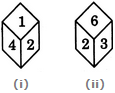A. 1 B. 2 C. 3 D. 6

Answer: Option B

Explanation:

From figures (i) and (ii), it is clear that 4, 1, 3 and 6 he adjacent to 2. Therefore, 5 must lie opposite 2. Thus, if 5 is at the top, then 2 must be at the bottom.

12.

If the total number of dots on opposite faces of a cubical block is always 7, find the figure which is correct.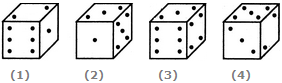A. Fig.1 B. Fig.2 C. Fig.3 D. Fig.4

Answer: Option B

Explanation:

Since the total number of dots on opposite faces is always 7, therefore, 1 dot appears opposite 6 dots, 2 dots appear opposite 5 dots and 3 dots appear opposite 4 dots.

13.

Two positions of a block are given below. When 1 is at the top, which number will be at the bottom?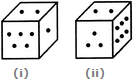A. 2 B. 3 C. 4 D. 6

Answer: Option D

Explanation:

Number 2 is common to the two positions of the dice. We assume the dice in fig. (ii) to be rotated so that 2 remains on the top face (i.e. face V as per activity 1) and the numbers 3 and 6 move to the faces hidden behind 5 and 1 respectively [in fig. (i)]. Then, clearly, 5 lies opposite 3 and 6 lies opposite 1. Hence, when 1 is at the top, then 6 will be at the bottom.

14.

What number is opposite 3 in the figure shown below? The given two positions are of the same dice whose each surface bears a number among 1, 2, 3, 4, 5 and 6.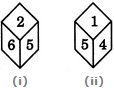A. 2 B. 4 C. 5 D. 6

Answer: Option C

Explanation:

From figures (i) and (ii), we conclude that 2, 6, 1 and 4 appear adjacent to 5. Therefore, 3 must appear opposite 5, Conversely, the number opposite 3 is 5.

15.

Two positions of a dice are shown below. Identify the number at the bottom when the top is '3'?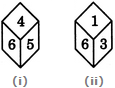A. 2 B. 4 C. 5 D. 6

Answer: Option C

Explanation:

The number 6 is common to both the positions of the dice. We assume the dice in fig. (ii) to be rotated so that 6 remains on the FR-LH face (i. e. on face IV as per activity 1) and the numbers 1 and 3 move to the faces hidden behind 4 and 5 respectively [in fig. (i)]. Then, 5 lies on FR-RH face (i.e. face I), 4 lies on Top face {i.e. face V), 3 lies on RR-LH face (i.e. face III) and 1 lies on Bottom face (i.e. face VI). Thus, 3 lies opposite 5. Hence, when the top is 3, then the number at the bottom is 5.

#### Current Affairs 2021

Interview Questions and Answers